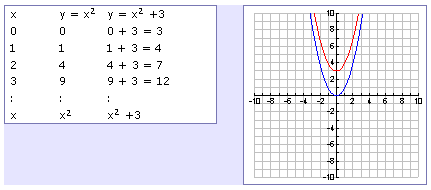Transformation
Up or Down

To understand the effects of k, compare the functions y = x2 and y = x2 + 3. The table below shows the pattern of values.By looking at both the table of values and the graph, k produces a vertical shift; in this case, k = 3 shifts the parabola up 3 units.

In function notation, to move a function up, add outside the function. The function f(x) + c is f(x) moved up c units. Moving the function down works the same way, the function f(x) - c is f(x) moved down c units.

Listen